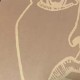# 一只菜鸡关于《Project NANO》的解谜记录

This article was published over 3年 ago. The information mentioned may be changed or developed.

この記事は 3年前に書かれたものです。記載された情報は変化した可能性があります。

### Real entry

<!--哦，我的老伙计，你怎么误打误撞到这里来了。-->
<!--认真告诉你，这里没东西，去研究下那个二维码吧。-->
<div class="inside-qr">
<p>哦，来了，我的老伙计。</p>
<p>都来到这里了，肯定不能太难是吧。</p>
<div class="hint">
<p>所以我要跟你打包票，这一关的题目真的<strong>只在这个二维码</strong>里面了。</p>
<p>要不，拆开看看？</p>
</div>
<img "https://cdn.jsdelivr.net/gh/hanlin-studio/[email protected]/vigen.png" />
<div class="hidden">
<a href="https://cdn.jsdelivr.net/gh/hanlin-studio/[email protected]/vigen.png">打开这个图片</a>
</div>
</div>

This is a 5*5 n-Puzzle. Please recover it.Scramble STEP: JNPSVWVGMKHGMEPAMEYJJXZ

4\times7!=2\,0160\text{ 种}

（至少比 25!=15511210043330985984000000={15\text{\footnotesize秭}5112\text{\footnotesize垓}1004\text{\footnotesize京}3330\text{\footnotesize兆}9859\text{\footnotesize亿}8400\text{\footnotesize万}} 种可能性要好得多。）

from PIL import Image
from itertools import permutations
from pyzbar.pyzbar import decode
p_0_0 = Image.open("0-0.png")
p_0_1 = Image.open("0-1.png")
p_0_3 = Image.open("0-3.png")
p_0_4 = Image.open("0-4.png")
p_1_0 = Image.open("1-0.png")
p_1_1 = Image.open("1-1.png")
p_1_2 = Image.open("1-2.png")
p_1_3 = Image.open("1-3.png")
p_1_4 = Image.open("1-4.png")
p_2_1 = Image.open("2-1.png")
p_3_0 = Image.open("3-0.png")
p_3_1 = Image.open("3-1.png")
p_3_3 = Image.open("3-3.png")
p_3_4 = Image.open("3-4.png")
p_4_0 = Image.open("4-0.png")
p_4_1 = Image.open("4-1.png")
p_4_3 = Image.open("4-3.png")
p_n_0 = Image.open("n-0.png")
p_n_1 = Image.open("n-1.png")
p_n_2 = Image.open("n-2.png")
p_n_3 = Image.open("n-3.png")
p_y_0 = Image.open("y-0.png")
p_y_1 = Image.open("y-1.png")
p_y_2 = Image.open("y-2.png")
p_y_3 = Image.open("y-3.png")
n = [p_n_0, p_n_1, p_n_2, p_n_3]
y = [p_y_0, p_y_1, p_y_2, p_y_3]
fixed = [
(0, 0, p_0_0),
(0, 1, p_0_1),
(0, 3, p_0_3),
(0, 4, p_0_4),
(1, 0, p_1_0),
(1, 1, p_1_1),
(1, 2, p_1_2),
(1, 3, p_1_3),
(1, 4, p_1_4),
(2, 1, p_2_1),
(3, 0, p_3_0),
(3, 1, p_3_1),
(3, 3, p_3_3),
(3, 4, p_3_4),
(4, 0, p_4_0),
(4, 1, p_4_1),
(4, 3, p_4_3),
]
left = [(0, 2), (2, 0), (2, 2), (2, 3), (2, 4), (3, 2), (4, 2)]
d = 20
def join_pic(yy, ns):
dest: Image = Image.new("RGB", (d*7, d*7), (255,255,255))
for x, y, img in fixed:
dest.paste(img, (d * (y + 1), d * (x + 1)))
for coord, img in zip(left, ns):
x, y = coord
dest.paste(img, (d * (y + 1), d * (x + 1)))
dest.paste(yy, (d*5, d*5))
return dest
def gen_perm():
for i in y:
ii = [j for j in y if j is not i]
ii.extend(n)
for j in permutations(ii):
yield (i, j)

for y, ns in gen_perm():
p = join_pic(y, ns)
v = decode(p)
if v:
print(v)

python3 test.py
[Decoded(data=b'https://clang.pp.ua/c1An9', type='QRCODE', rect=Rect(left=20, top=20, width=100, height=100), polygon=[Point(x=20, y=119), Point(x=120, y=120), Point(x=119, y=20), Point(x=21, y=21)])]

RULDDDRRURDRULLLULUUREV

• R: right = 右
• U: up = 上
• D: down = 下
• L: left = 左
• REV 提示「Reverse」，就是反转的意思。具体怎么反转的我也没太仔细研究。（毕竟是暴破出来的。）

（感谢群友 @MiaQAQ 的指点）

### Clang

<title>./.../.-./---/--</title>
<!-- ... -->
<div id="not-here">
<p class="what-is-this">这里 和那 里或 许没 什么 关系</p>
<p><code>1T1023298N757Q13142N10271Q1T1029</code></p>
<p>或许你需要先看看，嗯，标题。</p>
<p class="what-is-this">9432bc3b.log</p>
</div>

「日志」内容：

ZeroMe Logs | Mar 27, 2020.
undefined

[时序碎片：31 31]
[解锁 丢失的密钥：whereisthefilter]

• 标题 ./.../.-./---/-- 是摩尔斯电码的 ESROM（Morse 反过来写）
• 「这里 和那 里或 许没 什么 关系」→两字一组？
• 1T1023298N757Q13142N10271Q1T1029：不明。
• whereisthefilter：不是答案，用途不明

「门上插刀」似乎是出题者杜撰出来的一个「梗」。

，发表于

## 评论

### 《“一只菜鸡关于《Project NANO》的解谜记录”》 有 2 条评论

1.大学学的信息安全，就像是其他专业有acm，每年学长都会给我们准备ctf的题。
回想起来当时和同学通宵做题抢分，真是有意思。
一转眼都毕业三年了。

2. […] 之前写过了一篇选手视角的题解，亦可以作为参考：一只菜鸡关于《Project NANO》的解谜记录 […]